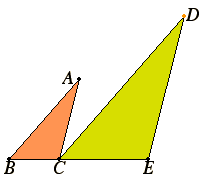# Proposition 32

If two triangles having two sides proportional to two sides are placed together at one angle so that their corresponding sides are also parallel, then the remaining sides of the triangles are in a straight line.

Let ABC and DCE be two triangles having the two sides AB and AC proportional to the two sides DC and DE, so that AB is to AC as DC is to DE, and AB parallel to DC, and AC parallel to DE.

I say that BC is in a straight line with CE.I.29

Since AB is parallel to DC, and the straight line AC falls upon them, therefore the alternate angles BAC and ACD equal one another.

For the same reason the angle CDE also equals the angle ACD, so that the angle BAC equals the angle CDE.

VI.6

And, since ABC and DCE are two triangles having one angle, the angle at A, equal to one angle, the angle at D, and the sides about the equal angles proportional, so that AB is to AC as DC is to DE, therefore the triangle ABC is equiangular with the triangle DCE. Therefore the angle ABC equals the angle DCE.

But the angle ACD was also proved equal to the angle BAC, therefore the whole angle ACE equals the sum of the two angles ABC and BAC.

Add the angle ACB to each. Therefore the sum of the angles ACE and ACB equals the sum of the angles BAC, ACB, and CBA.

I.32

But the sum of the angles BAC, ABC, and ACB equals two right angles, therefore the sum of the angles ACE and ACB also equals two right angles.

I.14

Therefore with a straight line AC, and at the point C on it, the two straight lines BC and CE not lying on the same side make the sum of the adjacent angles ACE and ACB equal to two right angles. Therefore BC is in a straight line with CE.

Therefore, if two triangles having two sides proportional to two sides are placed together at one angle so that their corresponding sides are also parallel, then the remaining sides of the triangles are in a straight line.

Q.E.D.

## Guide

The corresponding sides mentioned in the statement of the proposition are supposed to be directed in the same direction, even though that is not explicitly stated.

This proposition is used in the proof of proposition XIII.17 which inscribes a regular dodecahedron in a sphere.